# Rounding Times Down to a Specified Time Increment in Microsoft Excel 2010

In this article, we will learn how toround the times down to a specified time increment.We will use a combination of“TIME”, “HOUR”, “MINUTE” and “FLOOR” functionsin Microsoft Excel 2010.

FLOOR: - This function is used to round a number down to the nearest multiple of significance.

Syntax of “FLOOR” function:=FLOOR (number, significance)

Example:Cell A2 contains the number 456.25

=FLOOR (A2, 0.75), function will return 456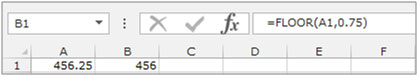HOUR: This function is used to return the hour as a number from 0 (12:00 AM) to 23 (11:00 PM).

Syntax of “HOUR” function:          =HOUR(serial_number)

For Example:Cell A1 contains 15:00 (3:00 PM).

• Write the formula in cell B1.
• =HOUR(A1), andpress Enter on your keyboard.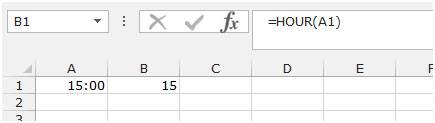MINUTE: This function is used to return the minute a number from 0 to 59.

Syntax of “MINUTE” function:      =MINUTE(serial_number)

For Example:Cell A1 contains 15:10 (3:10 PM).

• Write the formula in cell B1.
• =MINUTE(A1), and press Enter on your keyboard.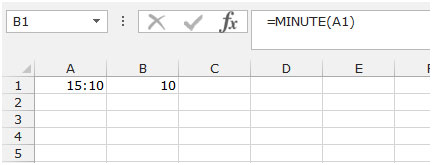TIME: -It converts hours, minutes, and seconds given as numbers to an Excel serial number, formatted with a time format.

Syntax: =TIME(hour,minute,second)

hour: A number from 0 to 23 representing hour

minute: A number from 0 to 59 representing minute

second: A number from 0 to 59 representing second

Let us take an example:

• We have hours listed in column A & minutes in column B
• We want a function that will combine both of them & return Time.
• The function in cell C2 would be =TIME(A2,B2,0)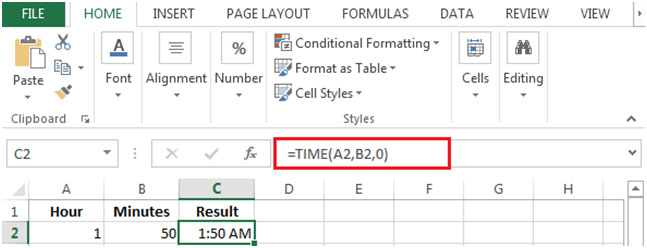Let’s take an example to understand how we can round times down to a specified time increment.

We have a time list formatted as “hh:mm:ss” in column A. Now, we want to round down to a specified time increment.Then we need to follow below given steps:-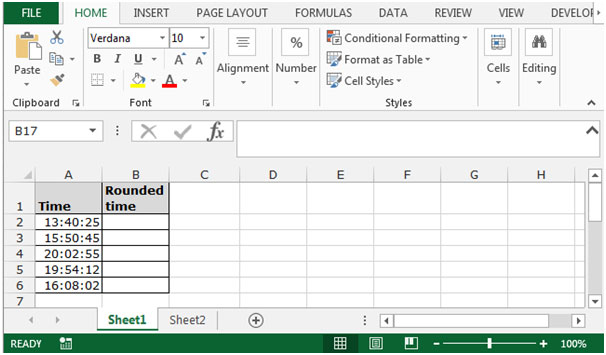• Write the formula in cell B2.
• =TIME(HOUR(A2),FLOOR(MINUTE(A2),15),0)
• The function will round the times down to a specified time increment.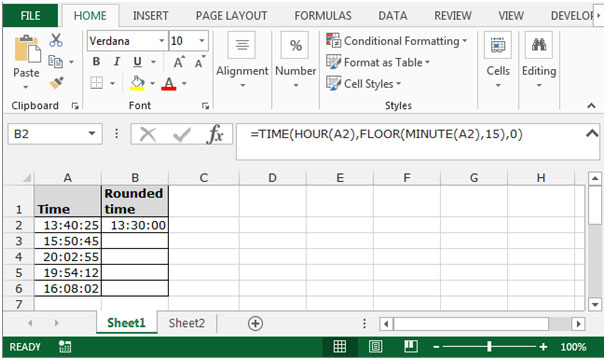• Copy the same formula by pressing the keyCtrl+C and paste in the range B3:B6 by pressing the key Ctrl+V on your keyboard.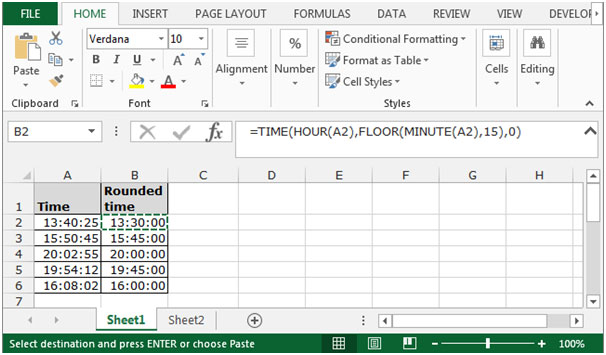This is the way we can round the times down to a specified time increment in Microsoft Excel.

1.2.